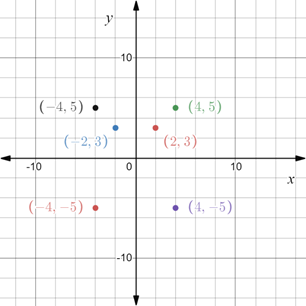# To graph : The points ( 2 , 3 ) , ( − 2 , 3 ) , ( 4 , 5 ) , ( 4 , − 5 ) , ( − 4 , 5 ) , ( − 4 , − 5 ) in a coordinate plane.### Precalculus: Mathematics for Calcu...

6th Edition
Stewart + 5 others
Publisher: Cengage Learning
ISBN: 9780840068071### Precalculus: Mathematics for Calcu...

6th Edition
Stewart + 5 others
Publisher: Cengage Learning
ISBN: 9780840068071

#### Solutions

Chapter 1.8, Problem 7E
To determine

## To graph: The points (2,3),(−2,3),(4,5),(4,−5),(−4,5),(−4,−5) in a coordinate plane.

Expert Solution

### Explanation of Solution

Given information:

The points, (2,3),(2,3),(4,5),(4,5),(4,5),(4,5) .

Graph:

Toplot the points (2,3),(2,3),(4,5),(4,5),(4,5),(4,5) in the coordinate plane.

Construct a Cartesian plane with vertical axis as y-axis and horizontal axis as x-axis.

Marks the numbers on both the axes.

Plot the points (2,3),(2,3),(4,5),(4,5),(4,5),(4,5) .Interpretation:

The Cartesian plane is divided into four quadrant. Numbers on right of zero are positive, on left of zero are negative. Numbers above zero on vertical line are positive and numbers below 0 are negative. Each pair of point in the coordinate plane is represented as an ordered pair (x,y) where first element is the x-coordinate and second element is the y-coordinate. The point (x,y) denote it is x units on x-axis and y units on y −axis and marked accordingly.

### Have a homework question?

Subscribe to bartleby learn! Ask subject matter experts 30 homework questions each month. Plus, you’ll have access to millions of step-by-step textbook answers!